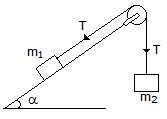Last updated: August 14, 2020

1. The potential energy of a vertically raised body is __________ the kinetic energy of a vertically falling body.
2. In ideal machines, mechanical advantage is __________ velocity ratio.
3. Which of the following is a scalar quantity?
4. The principle of transmissibility of forces states that, when a force acts upon a rigid body, its effect is
5. The loss of kinetic energy during elastic impact is zero.
6. Non-coplaner concurrent forces are those forces which
7. If a given force (or a given system of forces) acting on a body __________ the position of the body, but keeps it in equilibrium, then its effect is to produce internal stress in the body.
8. The point, through which the whole weight of the body acts, irrespective of its position, is known as
9. The periodic time of one oscillation for a simple pendulum is(where l = Length of the pendulum.)
10. The maximum efficiency of a lifting machine is (m= coefficient of friction, V.R= velocity ratio)
11. A differential pulley block has larger and smaller diameters of 100 mm and 80 mm respectively. Its velocity ratio is
12. A block of mass m1, placed on an inclined smooth plane is connected by a light string passing over a smooth pulley to mass m2, which moves vertically downwards as shown in the below figure. The tension in the string is13. The moment of inertia of a square of side a about its diagonal is
14. The periodic time of a particle with simple harmonic motion is __________ proportional to the angular velocity.
15. A lift moves downwards with an acceleration of 9.8 m/s2. The pressure exerted by a man on the floor of the lift is zero.
16. The motion of a particle around a fixed axis is
17. If three forces acting at a point are represented in magnitude and direction by the three sides of a triangle, taken in order, then the forces are in equilibrium.
18. The centre of gravity of a semi-circle lies at a distance of __________ from its base measured along the vertical radius(r).
19. The process of finding out the resultant force is called __________ of forces.
20. The maximum efficiency of a screw jack is
Engineering Mechanics MCQ 4
You got {{userScore}} out of {{maxScore}} correct
{{title}}
{{image}}
{{content}}